Question

A composite is produced from a titanium alloy reinforced with aligned silicon carbide fibres. The elastic moduli of titanium and silicon carbide are 118 GPa and 380 GPa respectively. The composite contains approximately 50% titanium and 50% fibres.

• a.Calculate the overall modulus of the composite, explaining your calculations and the assumptions you have made when it is loaded: Show all working and formula used:
• i.along the direction of fibre length
• ii.perpendicular to the fibre length.

(8 marks)

• b.Explain briefly why a material might show different yield behaviour in tension and compression when loaded cyclically.

Question a: Rule of mixtures is used to determine the modulii of composites in two directions.

The underlying assumptions:

1. There is a perfect bonding between fibers and matrix.

2. The fibers are uni directionally arranged in matrix.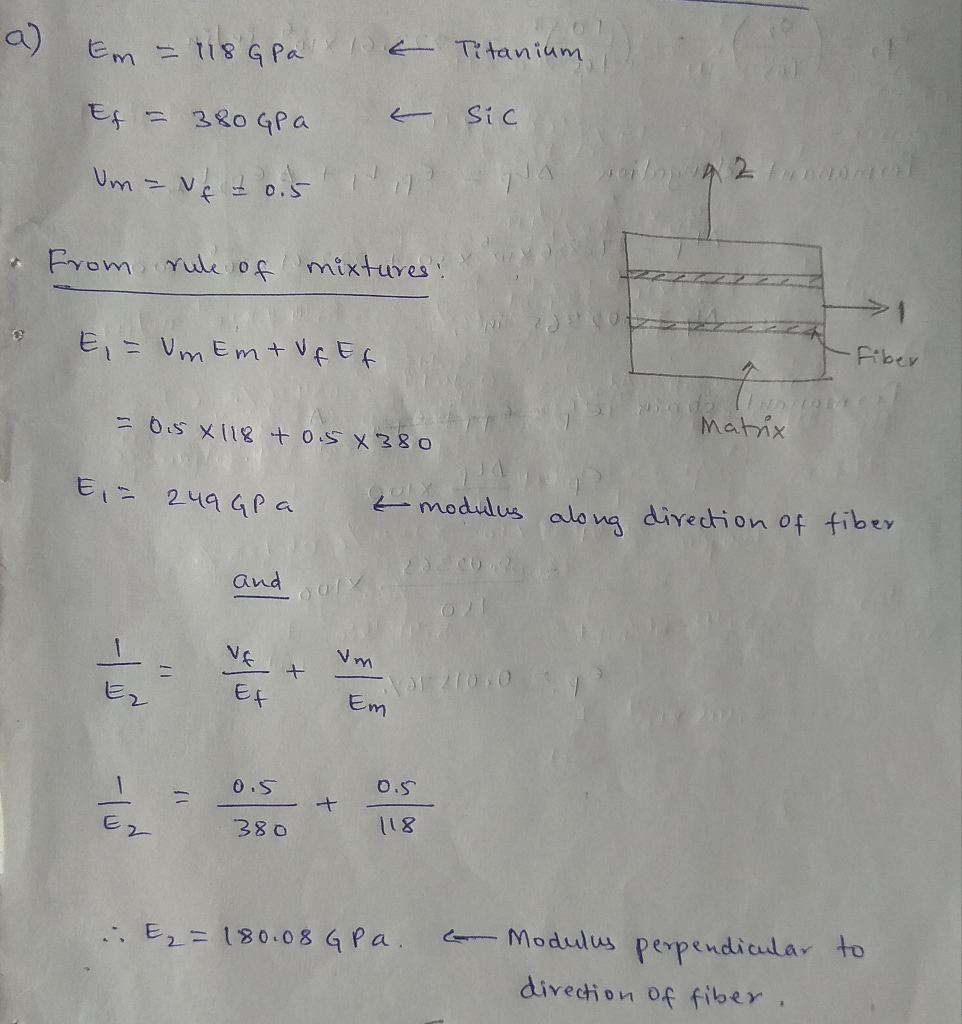Question b: The effect is known to be Bauschinger effect.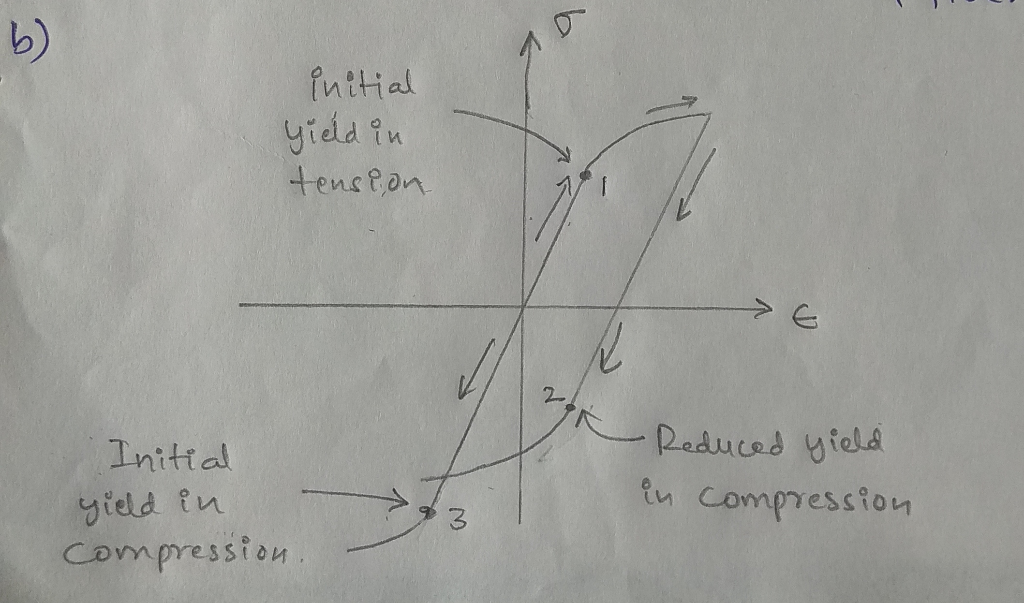It is a property of materials where the material's stress/strain characteristics change as a result of the microscopic stress distribution of the material. For example, an increase in tensile yield strength occurs at the expense of compressive yield strength. Titanium is well known to exhibit this phenomenon.

The figure above shows cyclic loading of a material. When the material is loaded in tension, it reaches its yield at point-1. However, when material is loaded beyond yield and the stress is reversed such that it is in compression, the yield point-2 occurs at lower stress. This value is lower than the yield stress when the material is loaded directly in compression (point-3).

#### Earn Coins

Coins can be redeemed for fabulous gifts.

Similar Homework Help Questions
• ### Question 1. A continuous and aligned fibre-reinforced composite material is to be produced consisting of 40...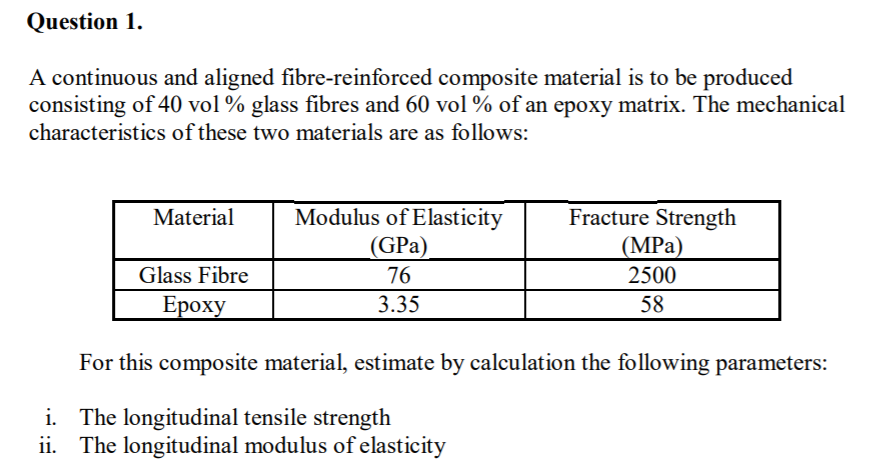Question 1. A continuous and aligned fibre-reinforced composite material is to be produced consisting of 40 vol % glass fibres and 60 vol % of an epoxy matrix. The mechanical characteristics of these two materials are as follows: MaterialModulus of Elasticity rcture Strength (GPa) (MPa) 2500 Glass Fibre 76 Epoxy 3.35 58 For this composite material, estimate by calculation the following parameters: i. The longitudinal tensile strength ii. The longitudinal modulus of elasticity

• ### Assume a composite made from a fibre volume fraction of 50% using uni directional, continuous fibres...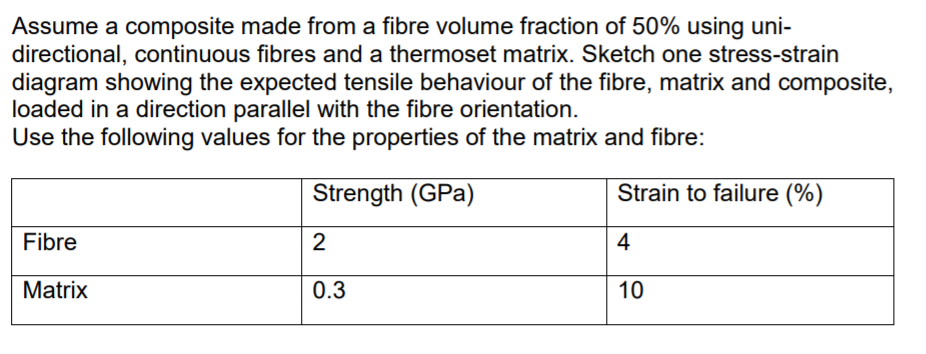Assume a composite made from a fibre volume fraction of 50% using uni directional, continuous fibres and a thermoset matrix. Sketch one stress-strain diagram showing the expected tensile behaviour of the fibre, matrix and composite, loaded in a direction parallel with the fibre orientation Use the following values for the properties of the matrix and fibre: Strain to failure (% Strength (GPa) Fibre 2 Matrix 0.3 10 Assume a composite made from a fibre volume fraction of 50% using uni...

• ### Q4 Acomposite consists of 65% polyester resin is reinforced by continuous and aligned glass fibers. The modulus elasticity of hardened resin is 4 0 GPa and the modulus elasticity of the ibers la...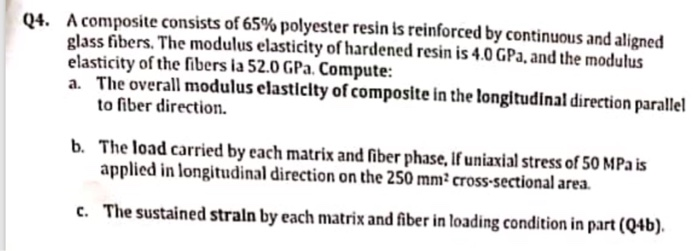Q4 Acomposite consists of 65% polyester resin is reinforced by continuous and aligned glass fibers. The modulus elasticity of hardened resin is 4 0 GPa and the modulus elasticity of the ibers la 52.0 GPa. Compute: a. The overall modulus elasticity of composite in the longitudinal direction parallel 04. to fiber direction b. The load carried by each matrix and iber phase, If uniaxial srss of 50 MPa is applied in longitudinal direction on the 250 mm2 cross-sectional area. c....

• ### versidad de Puerto Rico en Mayaguez matic bar AB, made of Titanium alloy a linearly elastic...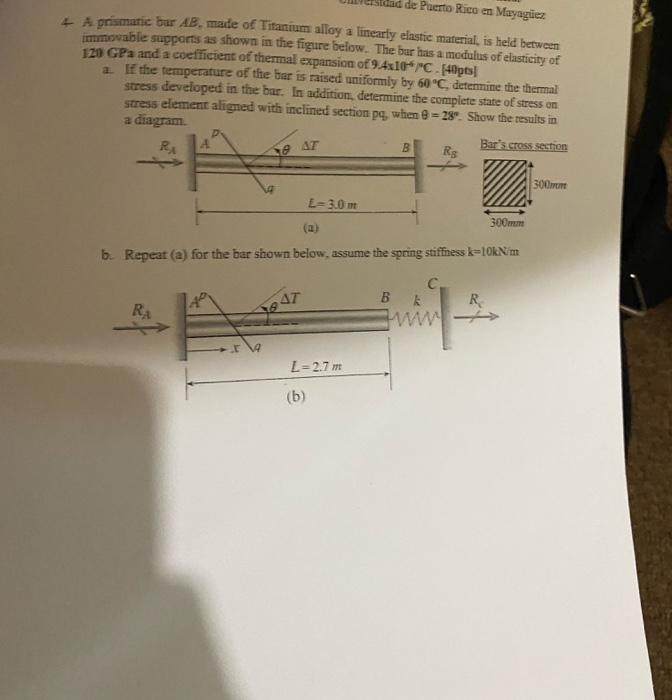versidad de Puerto Rico en Mayaguez matic bar AB, made of Titanium alloy a linearly elastic material is held between oyable supports as shown in the figure below. The bar has a modulus of elasticity of 120 GPa and a coefficient of thermal expansion of 9.4310-rc. [45pts] If the temperature of the bar is raised uniformly by 60 °C. determine the thermal stress developed in the bar. In addition, determine the complete state of stress on stress element aligned with...